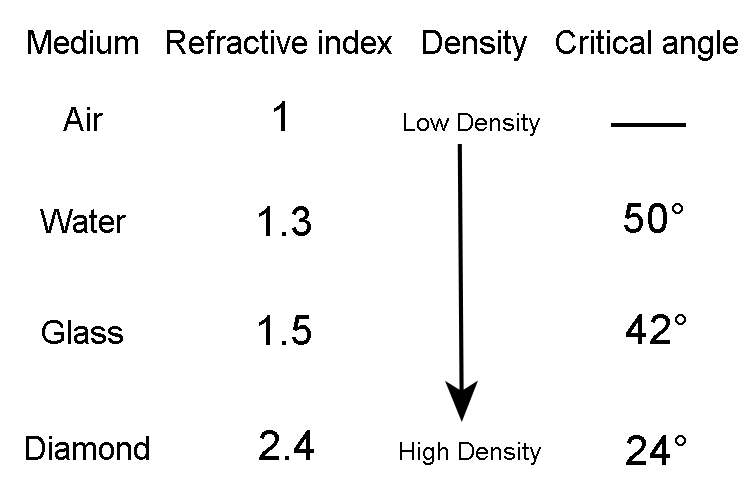Small critical angle

The smaller the critical angle the higher the refractive index.

Small critical angle rArr High refractive index

Water to air

Critical angle ~~50^circ  n=1.3Glass to air

Critical angle ~~42^circ  n=1.5Diamond to air

Critical angle ~~24^circ  n=2.4ConclusionNOTE: Low refractive number gives low density and large critical angle

High refractive number gives high density and small critical angle